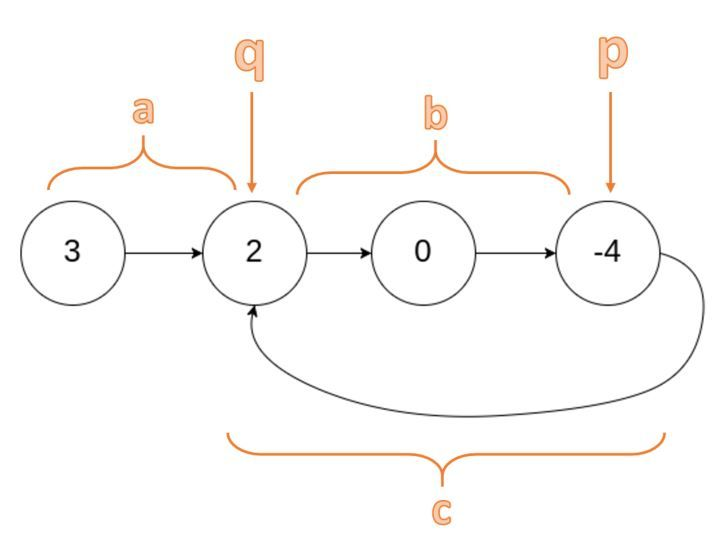### Given a linked list, find out if it has a cycle in it.

A linked list cycle looks like this:If there is no cycle in it, return `false`.

The idea is pretty simple. We just need a `slow` node which moves one step at a time, and a `fast` node which moves two steps at a time. If there is a cycle in the linked list, the `slow` node will eventually catch up with the `fast` node. Let's take a look at the solution:

``````    public boolean hasCycle(ListNode head) {

return false;
}

while(slow.next != null && fast.next != null && fast.next.next != null){
slow = slow.next;
fast = fast.next.next;
if(slow == fast){
return true;
}
}
return false;
}
``````

You just need to make sure that the `slow.next != null` and `fast.next.next != null`. When `slow == fast`, simply return `true`.

#### Follow up: if a cycle is found, can you return the node where the cycle begins?

In the first part, we can already determine whether a linked list has cycle or not. Now, we need to find out where the cycle begins. Let's find out!

Let's use the example above.When the `slow` and `fast` node meets at the point `p`, `slow` node has traveled `a + b` (2 steps) distance while `fast` node has traveled `a + b + c + b` (4 steps) distance.

Since the travel distance of `fast` node is twice the travel distance of `slow` node, we can safely conclude that `a + 2b + c = 2 (a + b)` which we then can simplify to `a = c`.

Once we can conclude that the travel distance `a` is actually the same as `c`, we can begin to move both `head` node and `slow` node forward, they will meet at point `q` where the cycle begins.

Here's the solution:

``````    public ListNode detectCycle(ListNode head) {

return null;
}

while(slow.next != null && fast.next != null && fast.next.next != null){

slow = slow.next;
fast = fast.next.next;
if(slow == fast){
while(curr != slow){
curr = curr.next;
slow = slow.next;
}
return curr;
}
}
return null;
}
``````

There we have it!

Post was published on , last updated on .

Like the content? Support the author by paypal.me!